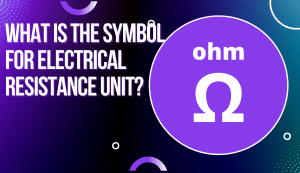# Electrical Resistance Unit: Everything You Need to KnowWhat is electrical resistance unit?

What are its types and symbols?

How do you calculate it?

Whether you’re here for a common crossword answer, or an expert electrician review, you will find your answer here!

The expert electrician of AC Electric will answer all of these questions and more.

Electrical resistance is a measure of how difficult it is for electricity to flow through a material. The higher the electrical resistance, the harder it is for current to flow.

There are three common types of electrical resistance unit:

• ohms (Ω)
• kilohms (kΩ)
• megohms (mΩ).

We will go over each type in detail below.

## What is the Symbol for Electrical Resistance Unit

When electrical resistance is written in mathematical equations, the symbol for electrical resistance unit is always Ω.

This is called an ohm.

An ohm is the SI unit of electrical resistance and is represented by the Greek letter omega.

## What is the equation for electrical resistance

The equation for electrical resistance is:

R = ρ × l / A

where:

ρ (rho) = resistivity

l = length

A = cross-sectional area

The resistivity of a material can be found in tables of electrical properties or measured with a multimeter.

The resistivity of common materials such as copper and aluminum can be looked up, but the resistivity of other materials must be measured.

To measure electrical resistivity, set your multimeter to the ohm setting and connect the probes to the material you are testing.

The electrical resistance of a material is affected by its temperature, so it is important to measure the resistivity at room temperature.

The electrical resistance of a conductor is also affected by its length and cross-sectional area.

The longer the conductor, the higher the electrical resistance.

This is because more electrons have to flow through the material.

The cross-sectional area also affects electrical resistance.

The larger the cross-sectional area, the lower the electrical resistance.

This is because there is a larger path for electrons to flow through.

Now that we know what an ohm is, let’s talk about kilohms and megohms.

## What is a Kilohm?

A kilohm (kΩ) is 1000 ohms and a megohm (mΩ) is 1000000 ohms.

These units are used when electrical resistance is very high.

For example, the electrical resistance of copper wire is about 16 Ω/km.

This means that for every kilometer of copper wire, there is 16 ohms of electrical resistance.

If we were to measure the electrical resistance of 1000 km of copper wire, it would be 16 kΩ.

The experts at AC Electric have years of experience dealing with electrical resistance unit.

We can help you choose the right type of electrical conductor for your needs and install it properly. Call us today for all your electrical needs!

## What is a Megohm?

If you work with electrical circuits, you might come across the unit of electrical resistance known as a megohm.

But what is a megohm?

A megohm is simply a unit of electrical resistance.

The electrical resistance of a material is how resistant it is to the flow of electricity.

The higher the resistance, the more difficult it is for electricity to flow through that material.

The standard unit for electrical resistance is called an ohm, named after German physicist Georg Simon Ohm.

One ohm is equal to the electrical resistance between two points when one volt (V) of potential difference causes a current of one ampere (A) to flow through the resistance.

So a megohm is just a million ohms, or a thousand kilohms.

Megohms are usually used to describe electrical insulation, meaning materials that have a high electrical resistance and don’t allow electricity to flow through them easily.

This makes them useful for electrical applications where you need to prevent electrical current from flowing, such as in electrical wiring.

The term “megohm” can also be used more generally to describe any extremely high electrical resistance.

So if you see someone talking about a megohm resistor or capacitor, they’re just referring to an electrical component with a very high level of resistance.

Hopefully, this gives you a better understanding of what megohms are and how they’re used.

Electrical resistance is an important property to consider when working with electrical circuits, so it’s good to have a basic understanding of the units involved.